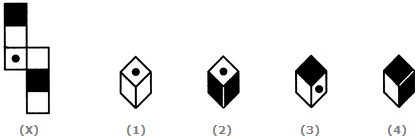# Non Verbal Reasoning - Cubes and Dice - Discussion

### Discussion :: Cubes and Dice - Section 1 (Q.No.10)

The sheet of paper shown in the figure (X) given on the left hand side, in each problem, is folded to form a box. Choose from amongst the alternatives (1), (2), (3) and (4), the boxes that are similar to the box that will be formed.

10.

Choose the box that is similar to the box formed from the given sheet of paper (X).[A]. 2 and 3 only [B]. 1, 3 and 4 only [C]. 2 and 4 only [D]. 1 and 4 only

Answer: Option B

Explanation:

The fig. (X) is similar to the Form V. So, when the sheet in fig. (X) is folded to form a cube, then the face bearing a dot lies opposite to one of the shaded faces. Therefore, the cube shown in fig. (2) which has both the shaded faces adjacent to the face bearing the dot, cannot be formed. Hence, the cubes shown in figures (1), (2) and (4) can be formed.

 Shubham said: (Aug 10, 2016) Option 1 can't possible since tow blank faces are adjacent to each other. But when we fold the cube these two blank faces will be opposite to each other.

 Georgin said: (Jul 21, 2018) Option 3 is false. If option 2 is false then same is applicable for 3 also. So, Answer should be 1 and 4.

 Kabyanjali Mahanta said: (Jun 7, 2021) Explain me in detail.

#### Post your comments here:

Name *:

Email   : (optional)

» Your comments will be displayed only after manual approval.

#### Current Affairs 2021

Interview Questions and Answers